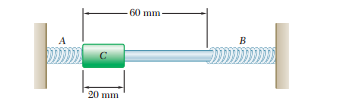### Find the period of the motion of the collar

Assignment Help Physics
##### Reference no: EM131429148

An 8-kg collar C can slide without friction on a horizontal rod between two identical springs A and B to which it is not attached. Each spring has a constant of 600 N/m. The collar is pushed to the left against spring A, compressing that spring 20 mm, and released in the position shown. It then slides along the rod to the right and hits spring B. After compressing that spring 20 mm, the collar slides to the left and hits spring A, which it compresses 20 mm. The cycle is then repeated. Determine

(a) the period of the motion of the collar,

(b) the position of the collar 1.5 s after it was pushed against spring A and released. (Note: This is a periodic motion, but not a simple harmonic motion.)#### Determine the period of vibration

A 30-lb uniform cylinder can roll without sliding on a 15°-incline. A belt is attached to the rim of the cylinder, and a spring holds the cylinder at rest in the position sh

#### Determine the mass of the disk

A circular disk of radius r = 0.8 m is suspended at its center C from wires AB and BC soldered together at B. The torsional spring constants of the wires are K1 = 100 N . m/

#### Find the period of small oscillations

A 10-lb uniform rod CD of length l =2.2 ft is welded at C to two elastic rods, which have fixed ends at A and B and are known to have a combined torsional spring constant K

#### Find the maximum velocity of end a of the rod

6-lb slender rod is suspended from a steel wire which is known to have a torsional spring constant K = 1.5 ft. lb/rad. If the rod is rotated through 180° about the vertical

#### Find the frequency of the resulting vibration

If corner B is given a small displacement and released, determine the frequency of the resulting vibration. Assume that each spring can act in either tension or compression

#### Determine the period of vibration

A 15-lb uniform cylinder can roll without sliding on an incline and is attached to a spring AB as shown. If the center of the cylinder is moved 0.4 in. down the incline and

#### Find centroidal radius of gyration of the connecting rod

A connecting rod is supported by a knife edge at point A; the period of its small oscillations is observed to be 1.03 s. Knowing that the distance ra is 150 mm, determine th

#### Determine the amplitude of the motion of the collar

9-lb collar can slide on a frictionless horizontal rod and is attached to a spring of constant 2.5 lb/in. It is acted upon by a periodic force of magnitude P = Pm sin vft, w

### Write a Review